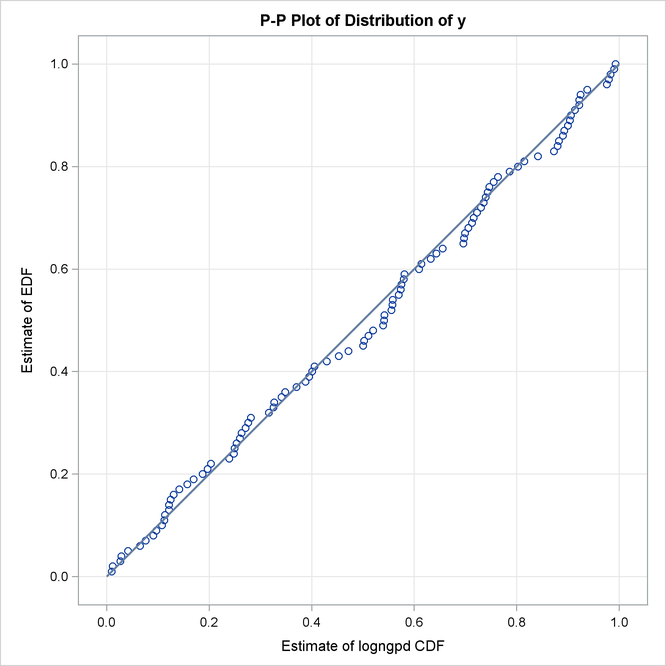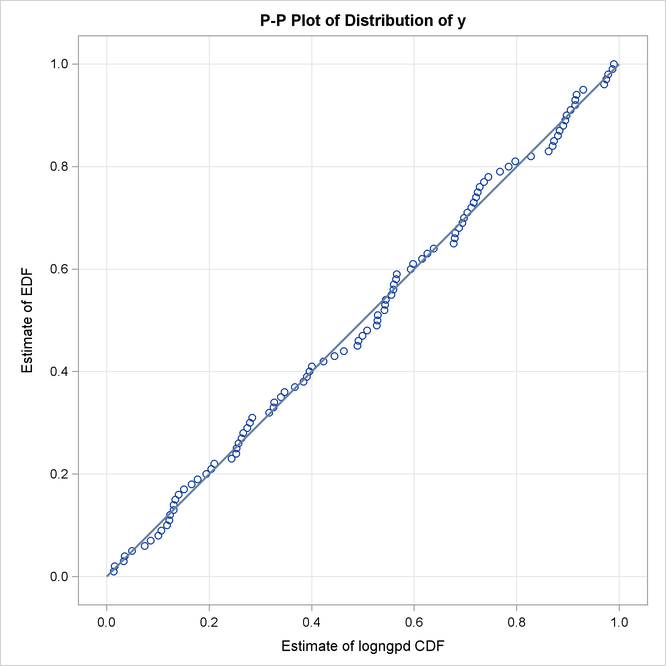# The SEVERITY Procedure

### Example 23.4 Estimating Parameters Using Cramér-von Mises Estimator

PROC SEVERITY enables you to estimate model parameters by minimizing your own objective function. This example illustrates how you can use PROC SEVERITY to implement the Cramér-von Mises estimator. Let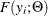denote the estimate of CDF atfor a distribution with parameters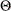, and let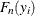denote the empirical estimate of CDF (EDF) atthat is computed from a sample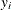,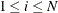. Then, the Cramér-von Mises estimator of the parameters is defined as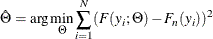This estimator belongs to the class of minimum distance estimators. It attempts to estimate the parameters such that the squared distance between the CDF and EDF estimates is minimized.

The following PROC SEVERITY step uses the Cramér-von Mises estimator to fit four candidate distribution models, including the LOGNGPD mixed-tail distribution model that was defined in Defining a Model for Mixed-Tail Distributions. The input sample is the same as is used in that example.

/*--- Set the search path for functions defined with PROC FCMP ---*/
options cmplib=(work.sevexmpl);

/*-------- Fit LOGNGPD model with PROC SEVERITY by using -------
-------- the Cramer-von Mises minimum distance estimator -------*/
proc severity data=testmixdist obj=cvmobj print=all plots=pp;
loss y;
dist logngpd burr logn gpd;

* Cramer-von Mises estimator (minimizes the distance *
* between parametric and nonparametric estimates)    *;
cvmobj = _cdf_(y);
cvmobj = (cvmobj -_edf_(y))**2;
run;



The OBJ= option in the PROC SEVERITY statement specifies that the objective function cvmobj should be minimized. The programming statements compute the contribution of each observation in the input data set to the objective function cvmobj. The use of keyword functions _CDF_ and _EDF_ makes the program applicable to all the distributions.

Some of the key results prepared by PROC SEVERITY are shown in Output 23.4.1. The "Model Selection" table indicates that all models converged. When you specify a custom objective function, the default selection criterion is the value of the custom objective function. The "All Fit Statistics" table indicates that LOGNGPD is the best distribution according to all the statistics of fit. Comparing the fit statistics of Output 23.4.1 with those of Output 23.3.1 indicates that the use of the Cramér-von Mises estimator has resulted in smaller values for all the EDF-based statistics of fit for all the models, which is expected from a minimum distance estimator.

Output 23.4.1: Summary of Cramér-von Mises Estimation

The SEVERITY Procedure

Input Data Set
Name WORK.TESTMIXDIST
Label Lognormal Body-GPD Tail Sample

Model Selection
Distribution Converged cvmobj Selected
logngpd Yes 0.02694 Yes
Burr Yes 0.03325 No
Logn Yes 0.03633 No
Gpd Yes 2.96090 No

All Fit Statistics
Distribution cvmobj -2 Log
Likelihood
AIC AICC BIC KS AD CvM
logngpd 0.02694 * 419.49635 * 429.49635 * 430.13464 * 442.52220 * 0.51332 * 0.21563 * 0.03030 *
Burr 0.03325   436.58823   442.58823   442.83823   450.40374   0.53084   0.82875   0.03807
Logn 0.03633   491.88659   495.88659   496.01030   501.09693   0.52469   2.08312   0.04173
Gpd 2.96090   560.35409   564.35409   564.47780   569.56443   2.99095   15.51378   2.97806

The P-P plots in Output 23.4.2 provide a visual confirmation that the CDF estimates match the EDF estimates more closely when compared to the estimates that are obtained with the maximum likelihood estimator.

Output 23.4.2: P-P Plots for LOGNGPD Model with Maximum Likelihood (Left) and Cramér-von Mises (Right) Estimators